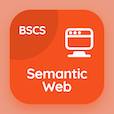Computer Science Courses

Digital Logic Design Exam Prep

Digital Logic Design Practice Test 68

The Book Subtraction With Complement Multiple Choice Questions and Answers (MCQs), subtraction with complement quiz answers PDF chapter 4-68 to study online courses, digital logic design tests. Study Binary Systems MCQ trivia questions, subtraction with complement Multiple Choice Questions (MCQ Quiz) for online college degrees. The App Subtraction With Complement MCQs e-Book PDF Download: subtraction with complement, analysis of asynchronous sequential logic, timing sequences, introduction to combinational logics, nand implementation test prep for information and communication technology.

The MCQ: X=1010100 and Y=1000011 using 2's complement X-Y is PDF, "Subtraction With Complement MCQs" App Android & iOS (Free) with 10110010, 1011101, 1001100, and 10001 choices for online degrees. Practice binary systems questions and answers, Google eBook to download free sample for associates in computer science.

## Logic Design: Subtraction With Complement MCQ Quiz

MCQ: X=1010100 and Y=1000011 using 2's complement X-Y is

A) 1011101
B) 10110010
C) 1001100
D) 10001

MCQ: Naming the states is done in

A) transition table
B) stable state
C) flow table
D) excitation table

MCQ: The word time signals can be generated utilizing a counter that counts the required number of

A) reset signals
B) Pluses
C) latches
D) flip-flops

MCQ: An integrated circuit with internal logic gates connected through electronic fuses is called

A) portable large device
B) portable logic device
C) programmable large device
D) programmable logic device

MCQ: NAND gate is the implementation o

A) NOT gates
B) OR gates
C) AND gates
D) XOR gates

### Mock Tests: Digital Logic Design Course Prep

The Apps: Digital Logic Design Quiz App, Semantic Web MCQs App, and DBMS MCQ App to download/install for Android & iOS devices. These Apps include complete analytics of real time attempts with interactive assessments. Download Play Store & App Store Apps & Enjoy 100% functionality with subscriptions!